# 基于Tecnomatix的四连杆机构运动原理介绍Kinematic Setup of Four-Bar Mechanism Based on Tecnomatix

• 全文下载: PDF(1032KB)    PP.33-38   DOI: 10.12677/MOS.2018.72005
• 下载量: 478  浏览量: 1,429

Based on the analysis of structure of four-bar mechanism, this paper establishes corresponding links in Tecnomatix. Kinematic joints are defined among the links. Through kinematic analysis, the functions of each joint are obtained. A program for generating kinematic functions is developed based on VBA.

1. 引言

2. 仿真软件中的四栏杆机构模型

2.1. 建立运动杆件

Tecnomatix (Process Simulate)中共同运动的部件集合称为杆件，给四连杆添加运动学的时候首先将不同部件及其附加结构分割成相应杆件。如图1，该四连杆机构应用于发动机罩盖板与车身的连接位置，在开启罩盖板时负责其开启角度的控制及与车身的支撑。其工作原理为：固定杆与车身相连，盖板平台通过螺钉与发动机罩盖板相连，抬起罩盖板过程中前后两支撑杆随之转动，最小角度定位螺钉与最大角度定位台阶分别用来限制罩盖板掀起的最小与最大角度。本文分析过程中以杆件1为固定杆件，设置杆件4为驱动杆件，通过计算各杆连接部位转动轴的角度关系，得到四连杆机构的运动规律。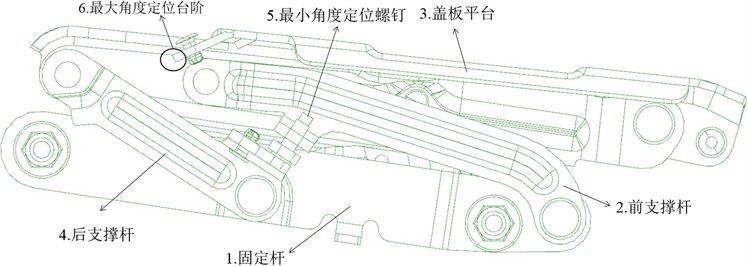1-固定杆，2-前支撑杆，3-盖板平台，4-后支撑杆，5-最小角度定位螺钉，6-最大角度定位台阶

Figure 1. Structure of four-bar mechanism

2.2. 建立运动副

3. 四连杆机构的运动学分析

3.1. 四连杆机构的机构类型Table 1. Link of four-bar mechanism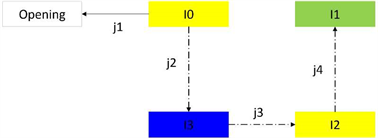Figure 2. Kinematical pairs and link of four-bar mechanism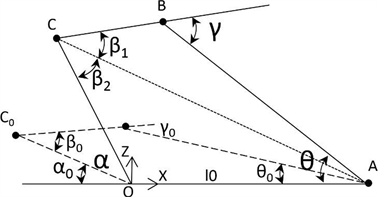Figure 3. Revolute mode diagram of four-bar mechanism

3.2. 四连杆机构运动学分析

${l}_{0},{l}_{1},{l}_{2},{l}_{3}$ 分别为图示杆件的长度：

${l}_{0}={x}_{1}$ (1)

${l}_{1}=\sqrt{{\left({x}_{1}-{x}_{2}\right)}^{2}+{z}_{2}^{2}}$ (2)

${l}_{2}=\sqrt{{\left({x}_{2}-{x}_{3}\right)}^{2}+{\left({z}_{2}-{z}_{3}\right)}^{2}}$ (3)

${l}_{3}=\sqrt{{x}_{3}^{2}+{z}_{3}^{2}}$ (4)

${\alpha }_{0}=-\mathrm{arctan}\frac{{z}_{3}}{{x}_{3}}$ (5)

${\beta }_{0}=\mathrm{arccos}\frac{{l}_{2}^{2}+{l}_{3}^{2}-\left({x}_{2}^{2}+{z}_{2}^{2}\right)}{2{l}_{2}{l}_{3}}$ (6)

${\gamma }_{0}=\text{π}-\mathrm{arccos}\frac{{l}_{1}^{2}+{l}_{2}^{2}-\left({\left({x}_{3}-{x}_{1}\right)}^{2}+{z}_{3}^{2}\right)}{2{l}_{1}{l}_{2}}$ (7)

$\alpha ={\alpha }_{0}+\Delta \alpha$ (8)

${l}_{1}^{2}+{l}_{2}^{2}-2{l}_{1}{l}_{2}\mathrm{cos}\left(\text{π}-\gamma \right)={l}_{0}^{2}+{l}_{3}^{2}-2{l}_{0}{l}_{3}\mathrm{cos}\left(\text{π}-\alpha \right)$ (9)

$\Delta \gamma =\mathrm{arccos}\frac{{l}_{0}^{2}+{l}_{3}^{2}-{l}_{1}^{2}-{l}_{2}^{2}+2{l}_{0}{l}_{3}\mathrm{cos}\left(\alpha \right)}{2{l}_{1}{l}_{2}}-{\gamma }_{0}$ (10)

$\left\{\begin{array}{l}{{x}^{\prime }}_{3}=-{l}_{3}\mathrm{cos}\alpha \\ {{z}^{\prime }}_{3}={l}_{3}\mathrm{sin}\alpha \end{array}$ (11)

${l}_{4}=\sqrt{{\left({l}_{3}\mathrm{sin}\alpha \right)}^{2}+{\left({x}_{1}+{l}_{3}\mathrm{cos}\alpha \right)}^{2}}$ (12)

$\Delta \beta ={\beta }_{1}+{\beta }_{2}-{\beta }_{0}=\mathrm{arccos}\frac{{l}_{2}^{2}+{l}_{4}^{2}-{l}_{1}^{2}}{2{l}_{2}{l}_{4}}+\mathrm{arccos}\frac{{l}_{3}^{2}+{l}_{4}^{2}-{l}_{0}^{2}}{2{l}_{3}{l}_{4}}-{\beta }_{0}$ (13)

4. 生成四连杆机构仿真运动函数的程序开发

4.1. 自定义运动副函数

Tecnomatix (Process Simulate)仿真软件对运动函数格式有严格要求，T表示转动副大小。由四连杆的运动关系，得到四连杆运动副的输入输出传递函数由分析可知j2的函数：

$T\left({j}_{2}\right)=T\left({j}_{1}\right)$ (14)

$T\left({j}_{4}\right)=\mathrm{arccos}\frac{{l}_{0}^{2}+{l}_{3}^{2}-{l}_{1}^{2}-{l}_{2}^{2}+2{l}_{0}{l}_{3}\mathrm{cos}\left(T\left({j}_{2}\right)+{\alpha }_{0}\right)}{2{l}_{1}{l}_{2}}-{\gamma }_{0}$ (15)

$\begin{array}{c}T\left({j}_{3}\right)=\mathrm{arccos}\frac{{l}_{2}^{2}+{\left({l}_{3}\mathrm{sin}\left(\text{π}-T\left({j}_{2}\right)-{\alpha }_{0}\right)\right)}^{2}+{\left({x}_{1}+{l}_{3}\mathrm{cos}\left(\text{π}-T\left({j}_{2}\right)-{\alpha }_{0}\right)\right)}^{2}-{l}_{1}^{2}}{2{l}_{2}\sqrt{{\left({l}_{3}\mathrm{sin}\left(\text{π}-T\left({j}_{2}\right)-{\alpha }_{0}\right)\right)}^{2}+{\left({x}_{1}+{l}_{3}\mathrm{cos}\left(\text{π}-T\left({j}_{2}\right)-{\alpha }_{0}\right)\right)}^{2}}}\\ \text{\hspace{0.17em}}\text{\hspace{0.17em}}+\mathrm{arccos}\frac{{l}_{3}^{2}+{\left({l}_{3}\mathrm{sin}\left(\text{π}-T\left({j}_{2}\right)-{\alpha }_{0}\right)\right)}^{2}+{\left({x}_{1}+{l}_{3}\mathrm{cos}\left(\text{π}-T\left({j}_{2}\right)-{\alpha }_{0}\right)\right)}^{2}-{l}_{0}^{2}}{2{l}_{3}\sqrt{{\left({l}_{3}\mathrm{sin}\left(\text{π}-T\left({j}_{2}\right)-{\alpha }_{0}\right)\right)}^{2}+{\left({x}_{1}+{l}_{3}\mathrm{cos}\left(\text{π}-T\left({j}_{2}\right)-{\alpha }_{0}\right)\right)}^{2}}}-{\beta }_{0}\end{array}$ (16)

4.2. 基于VBA的仿真函数生成程序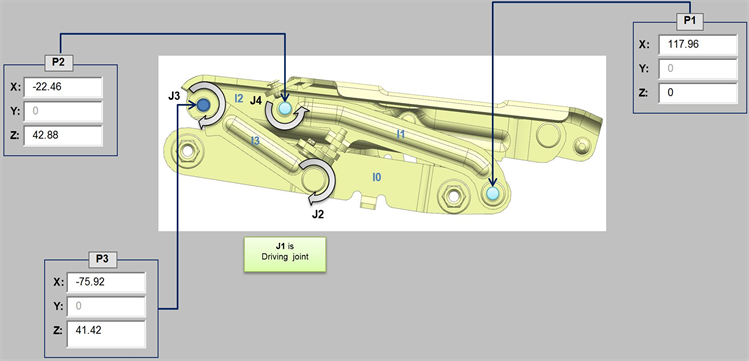Figure 4. Original coordinate interface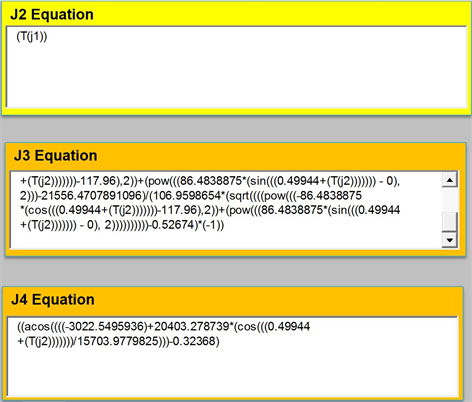Figure 5. Simulate function interface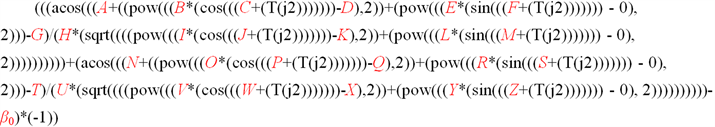5. 结束语

  王玲, 黄京. 基于Tecnomatix的气动焊枪运动学建立[J]. 机床与液压, 2015, 43(22): 1-3.